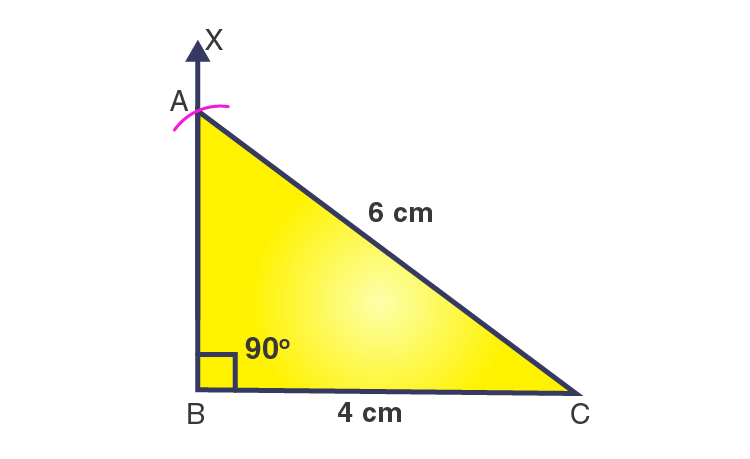# Construct a right-angled triangle whose hypotenuse is 6 cm long and one of the legs is 4 cm long

SolutionLet us consider ΔABC is a right angled triangle at ∠B = 90o

AC is hypotenuse = 6 cm … [given]

BC = 4 cm

The steps for construction are given below:

1. Draw a line segment BC = 4 cm.

2. From point B, draw a ray BX to making an angle of 90o i.e. ∠XBC = 90o.

3. Considering C as a center and radius 6 cm, draw an arc that cuts the ray BX at A.

4. Join AC.

Then, ΔABC obtained is the required right angled triangle.(0)(0)